By and large, there are two sorts of proof systems that people use (these days) when studying logic: natural deduction, and sequent calculus. I know of at least one other---Hilbert style---but it is older, and the above systems were invented due to dissatisfaction with Hilbert systems (for a programming analogy, Hilbert systems are like programming entirely with combinators (S, K, etc.), rather than a lambda calculus).

## Natural Deduction

Probably the best way to categorize the difference, for the purpose of where we're eventually going, is that natural deduction focuses on the ways to build proof terms up from their constituent parts. This comes in the form of introduction and elimination rules for the various propositions. For instance, the rules for conjunction are: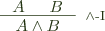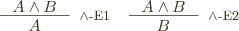This spartan style gets a bit annoying (in my opinion) for the hypothetical premises of the implication introduction, but this can be solved by adding contexts: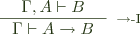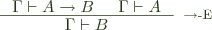This is the style most commonly adopted for presenting type theories, except we reason about terms with a type, rather than just propositions. The context we added for convenience above also becomes fairly essential for keeping track of variables: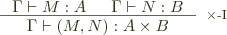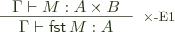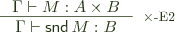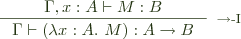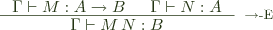As can be seen, all the rules involve taking terms from the premise and building on them in the conclusion.

## Sequent Calculi

The other type of system in question, sequent calculus, looks very similar, but represents a subtle shift in focus for our purposes (sequent calculi are a lot more obviously different when presenting classical logics). First, the inference rules relate sequents, which look a lot like our contextual judgments above, and I'll write them the same way. The difference is that not all rules operate on the conclusion side; some operate just on the context. Generally, introduction rules stay similar to natural deduction (and are called right rules), while elimination rules are replaced by manipulations of the context, and are called left rules. For pairs, we can use the rules: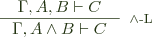We could also have two separate left rules: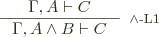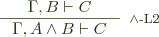But these two different sets are equivalent as long as we're not considering substructural logics. Do note, however, that we're moving from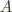on the top left to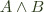on the bottom left, using the fact thatis sufficient to imply. That is, projections apply contravariantly to the left.

It turns out that almost no type theory is done in this style; natural deduction is far and away more popular. There are, I think, a few reasons for this. The first is: how do we even extend the left rules to type theory (eliminations are obvious, by contrast)? I know of two ways. The first is to introduce pattern matching into the contexts, so our left rule becomes: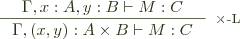This is an acceptable choice (and may avoid some of the pitfalls in the next option), but it doesn't gel with your typical lambda calculus. It's probably more suited to a pattern calculus of some sort (although, even then, if you want to bend your brain, go look at the left rule for implication and try to figure out how it translates into such a theory; I think you probably need higher-order contexts of some sort). Anyhow, I'm not going to explore this further.

The other option (and one that I've seen in the literature) is that left rules actually involve a variable substitution. So we come up with the following rule: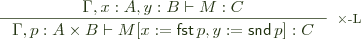And with this rule, it becomes (I think) more obvious why natural deduction is preferred over sequent calculus, as implementing this rule in a type checker seems significantly harder. Checking the rules of natural deduction involves examining some outer-most structure of the term, and then checking the constituents of the term, possibly in an augmented context, and which rule we're dealing with is always syntax directed. But this left rule has no syntactic correspondent, so it seems as though we must nondeterministically try all left rules at each step, which is unlikely to result in a good algorithm. This is the same kind of problem that plagues extensional type theory, and ultimately results in only derivations being checkable, not terms.

## The Type Class Connection

However, there are certain problems that I believe are well modeled by such a sequent calculus, and one of them is type class checking and associated dictionary translations. This is due mainly to the fact that the process is mainly context-directed term building, rather than term-directed type checking. As far as the type class algorithm goes, there are two interesting cases, having to do with the following two varieties of declaration:


class Eq a => Ord a where ...
instance (Eq a, Eq b) => Eq (a, b) where ...


It turns out that each of these leads to a left rule in a kind of type class sequent calculus: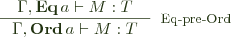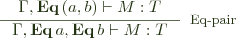That is:

1. if Eq a is a sufficient constraint for M : T, then the stronger constraint Ord a is also sufficient, so we can discharge the Eq a constraint and use Ord a instead.
2. We can discharge an Eq (a, b) constraint using two constraints, Eq a, Eq b together with an instance telling us how to do so. This also works for instances without contexts, giving us rules like: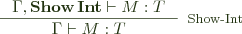Importantly, the type inference algorithm for type classes specifies when we should use these rules based only on the contexts we're dealing with. Now, these look more like the logical sequent rules, but it turns out that they have corresponding type theory-like versions when we consider dictionary passing: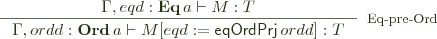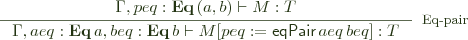And this kind of substituting into dictionary variables produces exactly the evidence passing translation we want.

Another way to look at the difference in feasibility is that type checking involves moving bottom-to-top across the rules; in natural deduction, this is always easy, and we need look only at the terms to figure out which we should do. Type class checking and dictionary translation moves from top-to-bottom, directed by the left hand context, and produces terms on the right via complex operations, and that is a perfect fit for the sequent calculus rules.

I believe this corresponds to the general opinion on those who have studied sequent calculi with regard to type theory. A quick search revealed mostly papers on proof search, rather than type checking, and type classes rather fall into that realm (they're a very limited form of proof search).